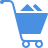Cart (0)

This is where data form a bell shape curve, that is, 50% of the distribution is above the mean and another 50% is below it. Also, in a normal distribution, the median is equal to the mean. Many statistical forecasting techniques assume that the historical data are normally distributed about the mean, and will yield poor results if this is not the case.

Previous Entry
Next Entry